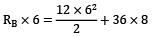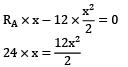Courses

# Test: SFD & BMD Level - 3

## 20 Questions MCQ Test Strength of Materials (SOM) | Test: SFD & BMD Level - 3

Description
This mock test of Test: SFD & BMD Level - 3 for Mechanical Engineering helps you for every Mechanical Engineering entrance exam. This contains 20 Multiple Choice Questions for Mechanical Engineering Test: SFD & BMD Level - 3 (mcq) to study with solutions a complete question bank. The solved questions answers in this Test: SFD & BMD Level - 3 quiz give you a good mix of easy questions and tough questions. Mechanical Engineering students definitely take this Test: SFD & BMD Level - 3 exercise for a better result in the exam. You can find other Test: SFD & BMD Level - 3 extra questions, long questions & short questions for Mechanical Engineering on EduRev as well by searching above.
QUESTION: 1

### Loading and shear force diagrams are given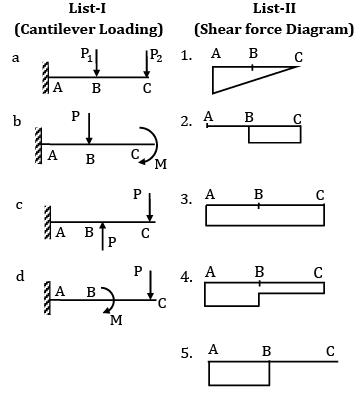Codes: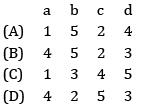Solution: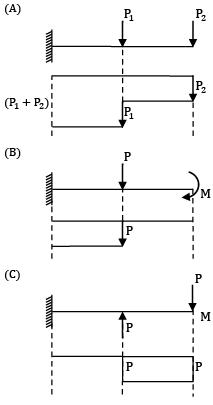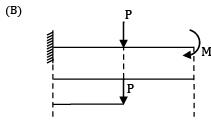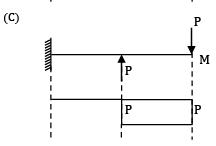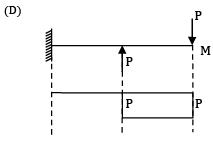QUESTION: 2

### The shear force diagram is shown above for a loaded beam. The corresponding bending moment diagram is represented by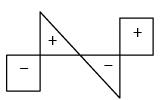Solution: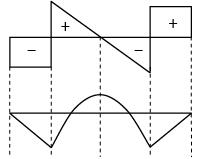When SF = Constant BM should have a linear variation.

When SF linearly varying BM should vary parabolically.

Both the conditions are satisfied for option (A).

QUESTION: 3

### Consider the following statements for a simply supported beam subjected to a couple of 10 kN-m at its mid-span.1. Bending moment is zero at the ends and maximum at the center2. Bending moment is constant over the entire length of the beam3. Shear force is constant over the entire length of the beam4. Shear force is zero over the entire length of the beam Which of the statements given above are correct?

Solution: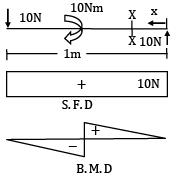QUESTION: 4

A simply supported beam of span ‘l’ subjected to a load linearly varying from zero at one end to w/m at the other, develops a maximum shear force of

Solution: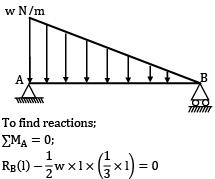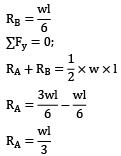The maximum shear force will occur at A.

QUESTION: 5

The left half of a simply supported beam of span “L” is loaded with u. d. l of w/meter run. The point of maximum B.M will occur at

Solution: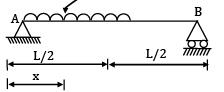We know RA + RB = wL / 2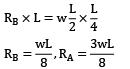For maximum B.M, S. F must be change sign shear force at

x = RA − wx = 0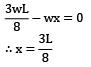QUESTION: 6

For the loaded beam as shown in the figure max B.M occurs at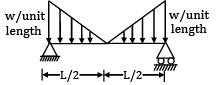Solution: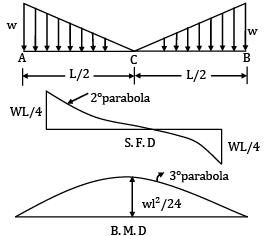∑Fy = 0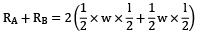= wl / 2

∴ RA = wL / 4

For maximum B.M, S.F must be changes sign Maximum B.M @ c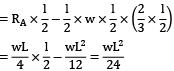QUESTION: 7

A load perpendicular to the plane of the handle is applied at the free end as shown in the given figure. The values of shear forces (S.F.), Bending Moment (B.M.) and torque at the fixed end of the handle have been determined respectively as 400N, 340Nm and 100 by a student. Among these values, those of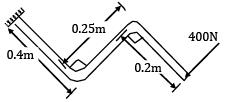Solution: S. F = 400N and BM = 400 × (0.4 + 0.2)

= 240Nm

Torque = 400 × 0.25 = 100Nm

QUESTION: 8

The shear force diagram of a loaded beam is shown in the following figure. The maximum bending moment of the beam is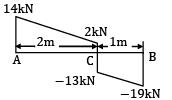Solution: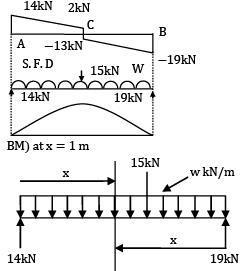15 + w x 3 = 14 + 19 = 33 ⇒ w = 6 kN/m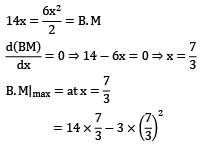= 16.33

QUESTION: 9

A beam AB, 8 m long hinged at end A and roller supported at end B carries a point load of 8 kN of crank ED = 1 m and uniformly distributed load of 5 kN/m over CB = 4 m. Then the bending moment where SF is zero is __________ kN-m.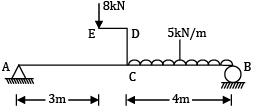Solution:

Load of 8 kN at E is equivalent to a load 8 kN at C and a CCW moment of 8 kN at C as shown in equivalent loading diagram as shown in figure.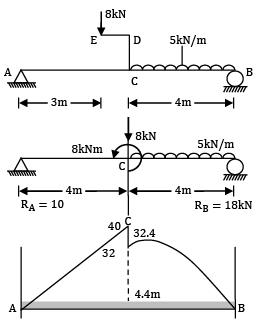8RB + 8 = 8 × 4 + 5 × 4 × (4 + 2)

= 32 + 20 × 6 = 152

RB = 15 kN

RA = 20 + 8 − 18 = 10 kN

BM diagram

MA = 0

MC = 10 × 4 = +40 kN

MC ′ = 40 − 8 = 32 kN M6 = 10 × 6 − 8 − 8 × 2 − 5 × 2 × 1

= 60 − 8 − 16 − 10 = 26 kN/m

M8 = 0

In portion CB

Fx = 10 − 8 − (x − 4) = 0

2 − 5(x − 4) = 0

2 = 5(x − 4)

x = 4.4 m

M = bending moment where SF = 0

= 10 × 4.4 − 8(4.4 − 4) − 8 − 5(4.4 − 4)(0.2)

= 44 − 3.2 − 8 − 5(0.4)(0.2)

= 32.8 − 0.4 = 32.4 kN/m

QUESTION: 10

A cantilever ABC, 5 m long is propped by a force of 8 kN at free end as shown in figure (a). It carries a UDL of 4 kN/m over BC = 3 m.

The point of contra-flexure is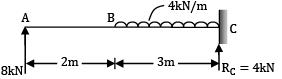Solution:

Reaction at C, RC = −8 + 12 = +4 kN (upwards) ↑

SF diagram: Portion AB(x = 0 to 2 m)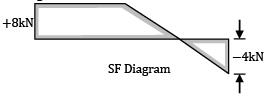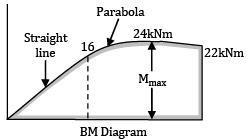FAB = +8 kN (constant)

(Taking upward forces on the left side of the section to be positive)

Portion BC (x = 2 to 5 m)

Fx = 8 − 4 (x − 2)

= 0 at x = 4 m

= −4 kN at x = 5

Figure (b) shows SF diagram.

BM diagram: Portion AB (x = 0 to 2 m)

Mx = +8x

= 0 at x = 0 m

= 16 kNm at x = 2 m

Portion BC (x = 2 to 5 m)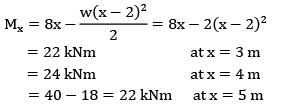There is no point of contra-flexure in the cantilever.

QUESTION: 11

Which one of the given bending moment diagrams correctly represents that of the loaded beam shown in figure?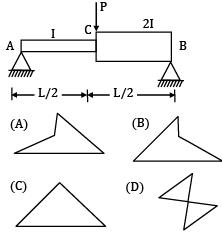Option~

(A)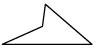(B)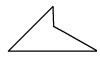(C)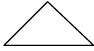(D)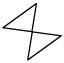Solution: Bending moment does not depend on moment of inertia.

QUESTION: 12

The intensity of loading on a simply supported beam of 5 meters span increases uniformly from 8 kN/m at one end to 16 kN/m at the other end. The magnitude of the maximum bending moment is __________ kN-m.

Reactions: Taking moments about the end A, we have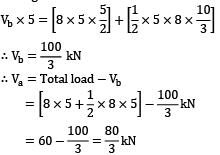Consider any section XX at a distance x from A Load intensity at the section XX

= 8 +x / 5 × 8 = 8 + 1.6x

S.F. Analysis S.F. at the section XX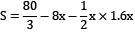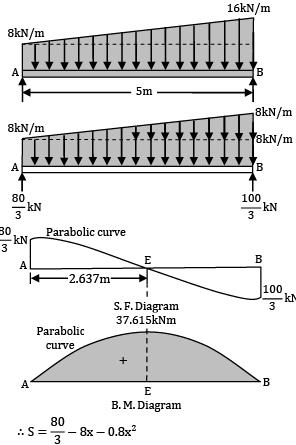The S.F. diagram follows a parabolic law At x = 0, i. e. , at A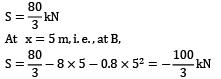Section of Zero Shear Equating the general expression for shear force to zero,

80 / 3 − 8x − 0.8x2 = 0

∴ 3x2 + 30x − 100 = 0

Solving, we get = 2.637 m.

B.M. Analysis

B.M. at any section XX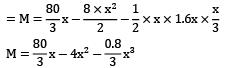The B.M. diagram follows a cubic law.

Maximum bending occurs at the section of zero shear, i.e., at a distance of 2.637 meters from A.

∴ Maximum bending moment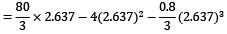= 37.615 kNm

Question_Type: 5

QUESTION: 13

A beam of uniform section 10 metres long carries a uniformly distributed load of 10 kN per metre over the whole length and a concentrated load of 10 kN at the right end. If the beam is freely supported at the left end, then the position of the second support so that maximum bending moment for the beam shall be as small as possible is __________ m.

Solution: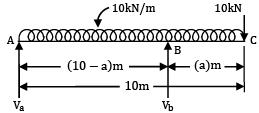Let the supports be at A and B so that the overhang BC = a metre ∴ AB = (10 − a)metre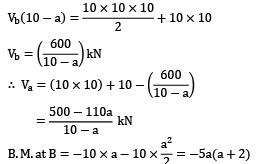Maximum positive B.M. will occur at a section in AB. Let this section be x metres from A.

∴ S. F. at this section = 0

∴ Va − 10x = 0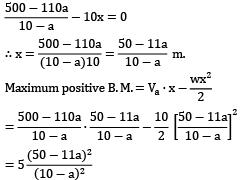For the condition that the maximum bending moment shall be as small as possible, the maximum positive bending moment and the hogging moment over the support should be numerically equal.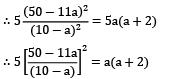Solving by trial and error, we get a = 2.33 m.

Maximum B.M. with the above value of a

= 5a(a + 2)

= 5 x 2.33(2.33 + 2)

= 50.44 kNm

Figure shows the beam showing the actual position of the supports.

SF and BM diagrams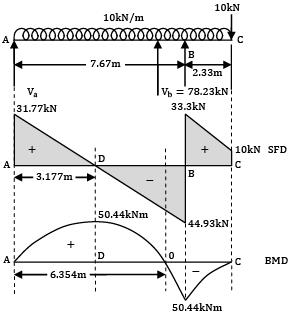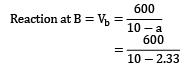78.23 kN

Reaction at A = Va = 110 − 78.23

= 31.77 kN.

Now the SF and BM diagram can be drawn easily.

Question_Type: 5

QUESTION: 14

A horizontal beam is simply supported at the ends and carries a uniformly distributed load of 10 kN/m between the supports placed 10 m apart. Anticlockwise moments of 150 kNm and 100 kNm are applied to the left and right ends of the beam at the supports. The point of contra-flexure from the right hand side support is __________m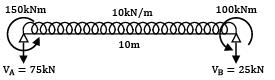Solution: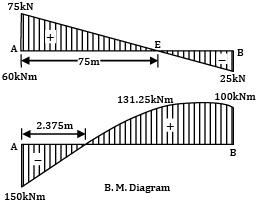Taking moments about the left end A,

Vb × 10 + 100 + 150 = 10 x 10 x 5

∴ Vb = 25 kN ↑

∴ Va = (10 × 10) − 25 = 75 kN ↑

S.F. Diagram. At any section distant x from A,

the shear force is given by,

S = 75 − 10x At x = 0, S = +75 kN,

At x = 10, S = −25 kN

Section of zero shear. Equating the general expression for shear force to zero,

75 − 10x = 0

∴ x = 7.5 m

B.M. Diagram. At any section distant x from the end A the Bending Moment is given by,

M = 75x − 5x2 − 150

At x = 0, M = −150 kNm At x = 10,

M = 750 − 500 − 150 = +100 kNm

At x = 7.5 m,

Mmax = 75 × 7.5 − 5 × 7.52 − 150

= +131.25 kNm

Point of contra-flexure. Equating the general expression for B.M. to zero,

75x − 5x2 − 150 = 0x2 − 15x + 30 = 0

Solving we get x = 2.375 m.

From right hand side support = 10 − 2.375

= 7.625m

Question_Type: 5

QUESTION: 15

A beam AB, 3 m long is hinged at end B to another beam BCDE, 5 m long as shown in figure (a). Beam AB carries a UDL of 2 kN/m over its full length, while beam BCDE carries a point load of 6 kNm, 1 m from C at D. The maximum shear force in the beam is __________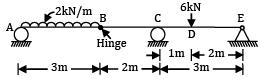Solution: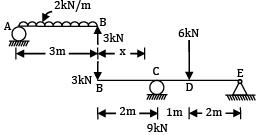Say reaction at B is RB, for beam AB this reaction will act load for beam BCDE.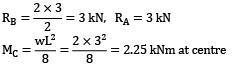Beam BCDE: Taking moments about point E.

6 x 2 + 3 x 5 = 3RC

Reaction, RC = 9 kN

Reaction, RE = 3 + 6 = 9 kN = 0 kN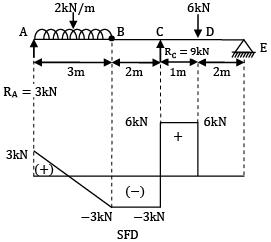Maximum shear force in the beam is 6 kN.

QUESTION: 16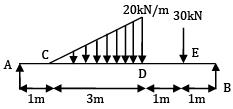Find maximum shear force.

Solution:

Vb x 6 = 30 × 5 + 1 / 2 x 3 x 20 x 3

Vb = 40 kN ↑

Total load applied = 30 + 1 / 2 x 3 x 20 = 60 kN

Va = 60 − 40 = 20 kN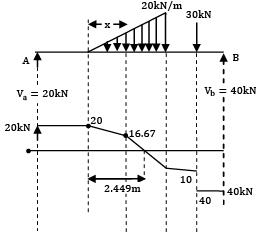Consider any section × in CD distance x from C.

Load intensity at the section x = 20x / 3

S. F at the section X = Sx = 20 − 1 / 2x − 20x / 3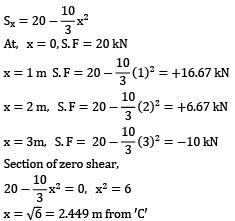S.F at any section in DE = 30 − 40 = −10 kN

S.F at any section in EB = −40 kN

QUESTION: 17

For the shown loading case the maximum bending moment will be __________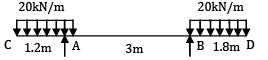Solution: Vb × 3 + 20 × 1.2 × 0.6 = 20 × 1.8(3 + 0.9)

3. Vb + 14.4 = 140.4

Vb = 42 kN

Va = (20 × 1.2) + (20 × 1.8) − 42 = 18 kN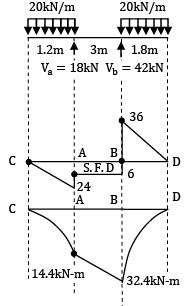BM at C = 0

B. M at A = −20 × 1.2 × 0.6

= −14.4 kNm

BM at B = −20 × 1.8 × 0.9

= −32.4 kNm

BM at D = 0

Question_Type: 5

QUESTION: 18

For the beam ABCDE shown in figure, the maximum bending moment is __________ kN-m.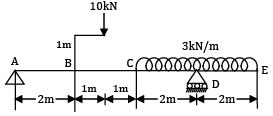Solution: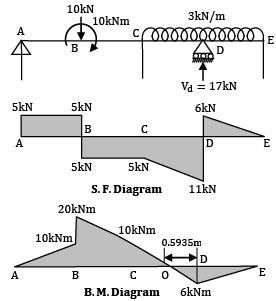The 10 kN load on the bracket is transmitted to B along with a clockwise couple of

10 × 1 = 10 kNm.

Vd × 6 = (10 × 3) + (3 × 4 × 6)

∴ Vd = 17 kN ↑ and

Va = 10 + (3 × 4) − 17 = 5 kN ↑

S.F. Analysis

S. F. at any section in AB = +5 kN

S. F. at any section in BC = +5 − 10 = −5 kN

S. F. at any section in CD,

distant x from C = S = 5 − 10 − 3x

∴ S = −5 − 3x

At x = 0, S = −5 kN, At x = 2,

S = −5 − 3 × 2 = −11 kN

S. F. at any section in DE,

distant x from E = S = 3x

At x = 0, S = 0 and at x = 2, S = +3 × 2 = +6 kN

The S. F. at D changes suddenly from

−11 kN to + 6 kN.

B. M Analysis B. M. at A= 0

B. M. on LHS of B = +5 × 2 = +10 kNm

B. M. on RHS of B = +10 + 10 = +20 kNm

B. M. at C = +5 × 4 − 10 × 2 + 10 = +10 Nm

B. M. at D = −3 × 2 × 1 = 6 kNm

B. M. at E = 0

Question_Type: 5

QUESTION: 19

SF diagram of a beam ABCD 6 m long, hinged at A and roller supported at C, is shown in figure. The distance of the point of contra-flexure from the left support is __________.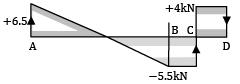Solution: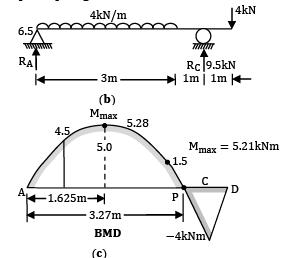From SF diagram Reaction at A, RA = 6.5 kN ↑

Reaction at C, RC = 5.5 + 4 = 9.5 kN ↑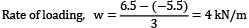Over AB = 3 m

Overhang, CD = 1 m

Load at point, D = 4.0 kN ↓

BM diagram

Position AB(x = 0 − 3 m)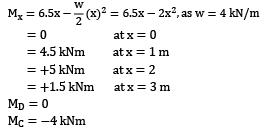M max.

SF in portion AB,

6.5 − 4(x) = 0 = SF

x = 1.625 m

Mmax = 6.5 × 1.625 − 2 × 1.6252

= 10.5625 − 5.28125

= 5.28 kNm

Point of contra-flexure Less in portion BC,

Mx = 6.5x − 4 × 3(x − 1.5)

Note that CG of UDL lies at 1.5 m from A

6.5x − 12(x − 1.5) = 0

6.5x − 12x + 18 = 0

18 = 5.5x

x = 3.27 m

Fig (c) shown BMD of the beam.

Question_Type: 5

QUESTION: 20

A beam ABC of segmental length AB=6m and BC = 2 m carries uniformly distributed load of intensity 12kN/m on AB and a point load of 36kN at C. Assuming simple supports at A and B, the point of contra flexure can be located at a section ___________m to the right of support A.

Solution: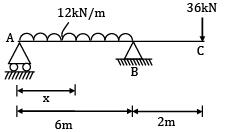RA + RB = 12 × 6 + 36 = 108kN